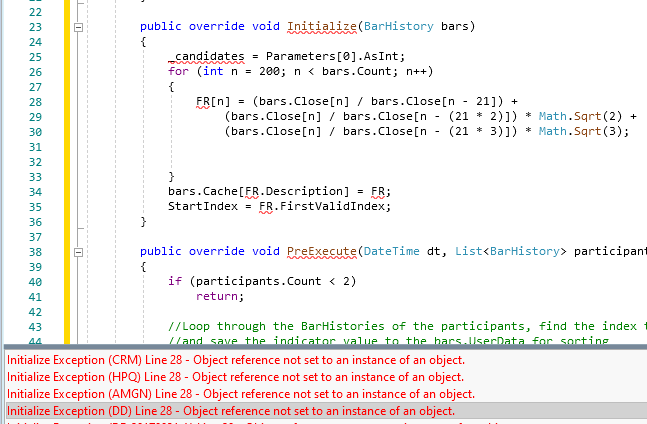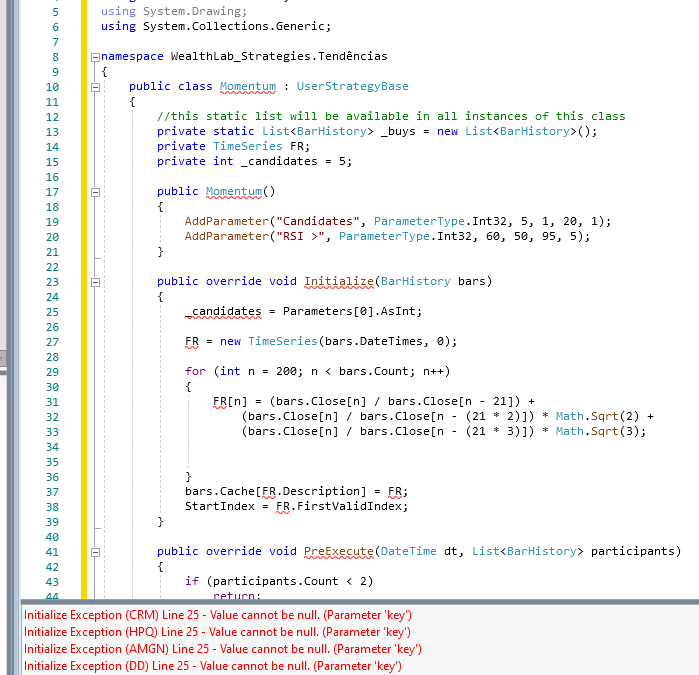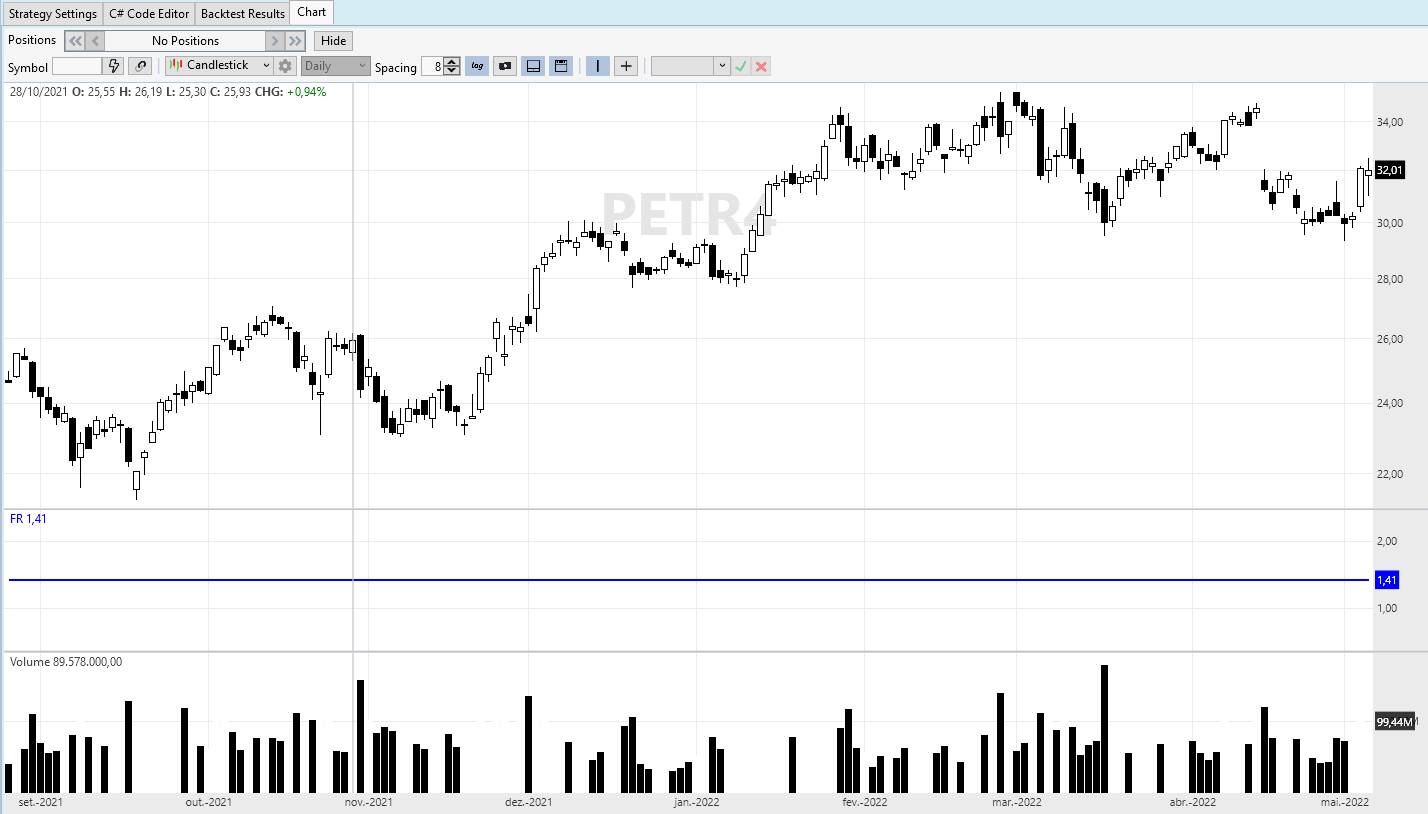Hello guys.

I need a code that do a price change comparison:

Current close divided by the close one month ago for all symbols in DataSet (%), then ranking the symbols top down. Then, assigns to each symbol a "Relative Force", from 100 to 0, according the highest variations.
After that, buys up to 5 positions (weighting by the highests % changes) but only if the "Relative Force" is bigger than 80 and RSI(5) is bigger than 60.

Exit positions conducting by EMA(9), if closes under EMA(9), exit at market.

I did a lot of research here on the forum, I found some discussions but I couldn't elaborate the system.

1
433
Solved

Bookmark

Sort
Hi Emilio,

It can be accomplished by creating a Rotation strategy in C# code. The code uses a monthly ROC (roughly) on daily data but it's up to you to come up with that Relative Force indicator.

```CODE:using WealthLab.Backtest;
using System;
using WealthLab.Core;
using WealthLab.Indicators;
using System.Drawing;
using System.Collections.Generic;

namespace WealthScript2
{
public class IndicatorRank7899 : UserStrategyBase
{
//this static list will be available in all instances of this class
private static List<BarHistory> _buys = new List<BarHistory>();
private ROC _roc;
private int _candidates = 5;

public IndicatorRank7899()
{
AddParameter("Candidates", ParameterType.Int32, 5, 1, 20, 1);
AddParameter("RSI >", ParameterType.Int32, 60, 50, 95, 5);
}

public override void Initialize(BarHistory bars)
{
_candidates = Parameters.AsInt;
_roc = ROC.Series(bars.Close, 30);
bars.Cache[_roc.Name] = _roc;
StartIndex = _roc.FirstValidIndex;
}

public override void PreExecute(DateTime dt, List<BarHistory> participants)
{
if (participants.Count < 2)
return;

//Loop through the BarHistories of the participants, find the index that corresponds to the DateTime dt,
//and save the indicator value to the bars.UserData for sorting
foreach (BarHistory bars in participants)
{
int idx = GetCurrentIndex(bars);
if (idx < 30) //30 days
{
bars.UserData = -1.0e10;      // change this to a negative value if you change the sort from higher to lower below
continue;
}
//monthly ROC ranked top down
bars.UserData = -(bars.Cache[_roc.Name] as TimeSeries)[idx];
}

//this Sorts from lower to higher.  For higher to lower, just change to .Sort(b, a)
participants.Sort((b, a) => b.UserDataAsDouble.CompareTo(a.UserDataAsDouble));

//Add the participants with the highest formula score to the _buys list
}

public override void Execute(BarHistory bars, int idx)
{
if (!HasOpenPosition(bars, PositionType.Long))
{
// only buy this one if it's in the _buys list
if (_buys.Contains(bars) && !double.IsNaN((double)bars.UserData))
{
//but only if the "Relative Force" is bigger than 80 and RSI(5) is bigger than 60.
if( (double)bars.UserData > 80)
if(RSI.Series(bars.Close,5)[idx] > Parameters.FindName("RSI >").AsInt)
}
}
else
{
//Exit positions conducting by EMA(9), if closes under EMA(9), exit at market.
if (bars.Close.CrossesUnder(EMA.Series(bars.Close,9),idx))
}
}
}
}```
0
Thanks Eugene, I will try to implement with some changes.

I have a doubt of how to "verify" if this type of strategy is really working properly, because with a strategy that manage only 1 asset per time, we simple look at the chart and analyze if it's entering and exiting correctly. With this kind of rotation strategy, I don't know how to compare the assets at given moment to understand if it's entering correctly. Do you have some advice for it?
0
The tools for checking and troubleshooting strategies can range from a simple WriteToDebugLog() call to using Visual Studio. For simplicity's sake you could start with the WriteToDebugLog output and assigning some value as the Position's entry signal name to look it up on the Positions tab. For more info please refer to the QuickRef.
1
ok, I will try. Thanks
0
Using your code, if I wish to replace the ROC with a custom calculation - let's say:

FR[n] = (Close[n] / Close[n - 30]) + (Close[n] / Close[n - (30* 2)]) + (Close[n] / Close[n - (30* 3)])

How could I make this work? The way I tried it I am getting an "object reference not set to an instance" error.

0
You have to change some lines to make it compile:
```CODE:
//private ROC _roc;
private TimeSeries _roc;

//_roc = ROC.Series(bars.Close, 30);
//bars.Cache[_roc.Name] = _roc;
_roc = your TimeSeries...
bars.Cache[_roc.Description] = _roc;

//bars.UserData = -(bars.Cache[_roc.Name] as TimeSeries)[idx];
bars.UserData = -(bars.Cache[_roc.Description] as TimeSeries)[idx];```
0
Hi Eugene, thanks for your help.

Probably I'm missing something related of how the language works. I'm tryng to implement the following "my TimeSeries":

```CODE: public class Momentum : UserStrategyBase
{
//this static list will be available in all instances of this class
private static List<BarHistory> _buys = new List<BarHistory>();
private TimeSeries FR;
private int _candidates = 5;

public Momentum()
{
AddParameter("Candidates", ParameterType.Int32, 5, 1, 20, 1);
AddParameter("RSI >", ParameterType.Int32, 60, 50, 95, 5);
}

public override void Initialize(BarHistory bars)
{
_candidates = Parameters.AsInt;
for (int n = 200; n < bars.Count; n++)
{
FR[n] = (bars.Close[n] / bars.Close[n - 21]) +
(bars.Close[n] / bars.Close[n - (21 * 2)]) * Math.Sqrt(2) +
(bars.Close[n] / bars.Close[n - (21 * 3)]) * Math.Sqrt(3);
}
bars.Cache[FR.Description] = FR;
StartIndex = FR.FirstValidIndex;```

It get compiled, but whe I run the backtest, I receive the following error code:I have been trying to implement something based on this answer I got from this post:
https://www.wealth-lab.com/Discussion/Create-a-cumulative-indicator-timeseries-7867

Could you tell me what I'm doing wrong?
0
You have to initialize the series first e.g.
`CODE:FR = new TimeSeries(bars.DateTimes,0);`
0
Now it changes the error:0
UPDATE 05/07/2022: fixed a bug

Try something like this instead:
```CODE:public override void Initialize(BarHistory bars)
{
_candidates = Parameters.AsInt;

_roc =    (bars.Close / (bars.Close >>21)) +
(bars.Close / (bars.Close>>(21*2))) * Math.Sqrt(2) +
(bars.Close / (bars.Close>>(21*3))) * Math.Sqrt(3);

bars.Cache[_roc.Description] = _roc;
StartIndex = _roc.FirstValidIndex;
}```
0
Nice Eugene, it seems that worked!

Now, I should change this part to fit the longer time of my TimeSeries, correct?

```CODE:foreach (BarHistory bars in participants)
{
int idx = GetCurrentIndex(bars);
if (idx < 30) //30 days
{
bars.UserData = -1.0e10;      // change this to a negative value if you change the sort from higher to lower below
continue;
}
//monthly ROC ranked top down
bars.UserData = -(bars.Cache[_roc.Description] as TimeSeries)[idx];```
0
Right. Maybe make it more adaptive with this:
`CODE:if (idx < StartIndex) //200 days`
0
Ok, I will try to test somehow if it's entering correctly. For now, thank you very much!
0
Hi Eugene

It took me quite a while to get a chance to check out the code, and looking at the results I think something is not working properly. When I investigated, I came up with the following situation (created just to understand how the syntax works):

I have written a simple code to plot an indicator that was being created similar to the code in the post, using shifting (bars.Close >>21). When I run it, it plots a constant timeseries. which totally explains the results I was noticing in the backtests. Any ideas on how to solve the problem?

```CODE:using WealthLab.Backtest;
using System;
using WealthLab.Core;
using WealthLab.Indicators;
using System.Collections.Generic;

namespace WealthScript4
{
public class MyStrategy : UserStrategyBase
{
private TimeSeries FR = new TimeSeries();

//create indicators and other objects here, this is executed prior to the main trading loop
public override void Initialize(BarHistory bars)
{
FR = (bars.Close / bars.Close >> 21) * Math.Sqrt(2);

PlotTimeSeries(FR, "FR", "FR_Pane", WLColor.Blue, PlotStyle.Line);
}

//execute the strategy rules here, this is executed once for each bar in the backtest history
public override void Execute(BarHistory bars, int idx)
{
if (!HasOpenPosition(bars, PositionType.Long))
{
}
else
{
//code your sell conditions here
}
}

//declare private variables below

}
}```0
Check your order of operations. You’re just dividing close by close, shifting the result (1) to the right, then multiplying by the square root of 2. So the result is the square root of 2. You’ll need parenthesis around the shift to give it precedence.
2
My bad, I coded that snippet on-the-fly and didn't check it. Glitch is right as always, here's what needs to be done:

```CODE://FR = (bars.Close / bars.Close >> 21) * Math.Sqrt(2);
FR = (bars.Close / (bars.Close >> 21)) * Math.Sqrt(2);```

I updated the code in post #10.
1
Oh man, what a huge difference a simple parenthesis can make!
Thank you both!

Trying to use WriteToDebugLog as Eugene suggested, I wrote the following (taken from some other post about ranking):

```CODE:public override void Execute(BarHistory bars, int idx)
{
string buySymbols = string.Join(", ", _buys.Select(x => x.Symbol));

if (!HasOpenPosition(bars, PositionType.Long))
{
// only buy this one if it's in the _buys list
if (_buys.Contains(bars) && !double.IsNaN((double)bars.UserData))
{
//but only if the "Relative Force" is bigger than 80 and RSI(5) is bigger than 60.
//if( (double)bars.UserData > 80)
if (RSI.Series(bars.Close, 5)[idx] > Parameters.FindName("RSI >").AsInt)
{
WriteToDebugLog(bars.DateString(idx) + "Compra: " + bars.Symbol + ": " + FR[idx]);
}
}
}
else```

This enabled me to check the symbols inside _buys. But I wonder if there is some way for me to check the "_roc" value of each component of _buys in each iteration (or something like that). This would help me to check if it is being bought correctly. I have tried a couple of things, but without success.
0
You're welcome. I think you're looking for this:
`CODE:WriteToDebugLog( (double)bars.UserData );`

BTW you may want to uncomment it in your code because it was commented in mine for debug purposes:
`CODE:if( (double)bars.UserData > 80)`

1
It worked perfectly!

This thread was a complete training course, thank you very much.
0
Glad to see you're up and running!
1
Hi Eugene and Glitch,

Just to complement, regarding the request there in post #1
QUOTE:
assigns to each symbol a "Relative Force", from 100 to 0, according to the highest variations

I included some stuff in the code to work with these percentiles:

I added a List to include all the symbols than are in the DataSet:
```CODE://Add the participants to calculate percentiles
_RFcalc.Clear();

Then calculate the percentiles according to position in "_buys" list:

```CODE:public override void Execute(BarHistory bars, int idx)
{
qtySymbols = _FRcalc.Count;

FRfinalPos = _FRcalc.FindIndex(x => x.UserDataAsDouble == FRseries[idx]);

FR = (qtySymbols - FRfinalPos)* 100 / qtySymbols;```

So, in the item that Eugene commented for Debug purposes in post #18, I have changed it and included a WriteToDebugLog as below:

```CODE: if (!HasOpenPosition(bars, PositionType.Long))
{
// only buy this one if it's in the _buys list
if (_buys.Contains(bars) && !double.IsNaN((double)bars.UserData))
{
//but only if the "Relative Force" is bigger than 80 and RSI(5) is bigger than 60.
if (RF > 80)
{
if (RSI.Series(bars.Close, 5)[idx] > Parameters.FindName("RSI >").AsInt)
{
string buySymbols = string.Join(", ", _buys.Select(x => x.Symbol));
string buyRF = string.Join(" RF: ",_buys.Select(y => y.UserData));
WriteToDebugLog(bars.DateString(idx) + Environment.NewLine +
" - Qty Symbols:: " + string.Format("{0:##00}", qtySymbols) + Environment.NewLine +
" - Symbol: " + bars.Symbol + ": " + string.Format("{0:0.000}", FRseries[idx]) + " || Buys: " + buySymbols + Environment.NewLine +
" - RF: " + buyRF + Environment.NewLine +
" - RF Pos: " + FRfinalPos + Environment.NewLine +
" - RF Value: " + RF + Environment.NewLine);
//WriteToDebugLog(bars.DateString(idx) + "Symbol: " + bars.Symbol + "       - MaxFR: " + maxFR);